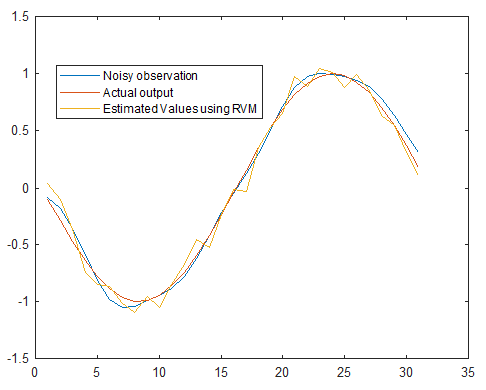Sale!

# MATLAB code for Relevance Vector Machine

19

## Description

Classical SVM can be considered as a linear regression problem. If we assume that the prior density function is a Gaussian distribution, then we can use Bayesian techniques.output of MATLAB code RVM## Reviews

There are no reviews yet.

SKU: 9RVMCDE7 Category: